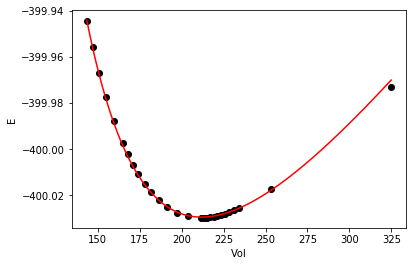## Using autograd in nonlinear regression

| categories: | tags: | View Comments

Table raw-data contains the energy as a function of volume for some solid material from a set of density functional theory calculations. Our goal is to fit the Murnaghan equation of state to this data. The model is moderately nonlinear. I have previously done this with the standard nonlinear regression functions in scipy, so today we will use autograd along with a builtin optimizer to minimize an objective function to achieve the same thing.

The basic idea is we define an objective function, in this case the summed squared errors between predicted values from the model and known values from our data. The objective function takes two arguments: the model parameters, and the "step". This function signature is a consequence of the built in optimizer we use; it expects that signature (it is useful for batch training, but we will not use that here). We use autograd to create a gradient of the objective function which the adam optimizer will use to vary the parameters with the goal of minimizing the objective function.

The adam optimizer function takes as one argument a callback function, which we call summary to print out intermediate results during the convergence. We run the optimizer in a loop because the optimizer runs a fixed number of steps on each call. We check if the objective function is sufficiently small, and if it is we break out.

import autograd.numpy as np

np.set_printoptions(precision=3, suppress=True)

# input data
Vinput = np.array([row for row in data])
Eknown = np.array([row for row in data])

def Murnaghan(pars, vol):
'''
given a vector of parameters and volumes, return a vector of energies.
equation From PRB 28,5480 (1983)
'''
E0, B0, BP, V0 = pars
E = E0 + B0 * vol / BP * (((V0 / vol)**BP) / (BP - 1.0) + 1.0) - V0 * B0 / (BP - 1.)
return E

def objective(pars, step):
"This is what we want to minimize by varying the pars."
predicted = Murnaghan(pars, Vinput)
# Note Eknown is not defined in this function scope
errors = Eknown - predicted
return np.sum(errors**2)

# Note i, N are not defined in this function scope
if step % N == 0:
print('step {0:5d}: {1:1.3e}'.format(i * N + step,
objective(pars, step)))

pars = np.array([-400, 0.5, 2, 210]) # The initial guess
N = 200 # num of steps to take on each optimization
learning_rate = 0.001
for i in range(100):
num_iters=N, callback=summary)
SSE = objective(pars, None)
if SSE < 0.00002:
print('Tolerance met.', SSE)
break
print(pars)

step     0: 3.127e+02
step   200: 1.138e+02
step   400: 2.011e+01
step   600: 1.384e+00
step   800: 1.753e-01
step  1000: 2.044e-03
step  1200: 1.640e-03
step  1400: 1.311e-03
step  1600: 1.024e-03
step  1800: 7.765e-04
step  2000: 5.698e-04
step  2200: 4.025e-04
step  2400: 2.724e-04
step  2600: 1.762e-04
step  2800: 1.095e-04
step  3000: 6.656e-05
step  3200: 3.871e-05
step  3400: 2.359e-05
('Tolerance met.', 1.5768901008364176e-05)
[-400.029    0.004    4.032  211.847]



There are some subtleties in the code above. One is the variables that are used kind of all over the place, which is noted in a few places. Those could get tricky to keep track of. Another is the variable I called learning_rate. I borrowed that terminology from the machine learning community. It is the step_size in this implementation of the optimizer. If you make it too large, the objective function doesn't converge, but if you set it too small, it will take a long time to converge. Note that it took at about 3400 steps of "training". This is a lot more than is typically required by something like pycse.nlinfit. This isn't the typical application for this approach to regression. More on that another day.

As with any fit, it is wise to check it out at least graphically. Here is the fit and data.

%matplotlib inline
import matplotlib
matplotlib.rc('axes.formatter', useoffset=False)
import matplotlib.pyplot as plt

plt.plot(Vinput, Eknown, 'ko', label='known')

vinterp = np.linspace(Vinput.min(), Vinput.max(), 200)

plt.plot(vinterp, Murnaghan(pars, vinterp), 'r-', label='predicted')
plt.xlabel('Vol')
plt.ylabel('E')The fit looks pretty good.

Table 1: Volume-Energy data for a solid state system.
volume energy
324.85990899 -399.9731688470
253.43999457 -400.0172393178
234.03826687 -400.0256270548
231.12159387 -400.0265690700
228.40609504 -400.0273551120
225.86490337 -400.0280030862
223.47556626 -400.0285313450
221.21992353 -400.0289534593
219.08319566 -400.0292800709
217.05369547 -400.0295224970
215.12089909 -400.0296863867
213.27525144 -400.0297809256
211.51060823 -400.0298110000
203.66743321 -400.0291665573
197.07888649 -400.0275017142
191.39717952 -400.0250998136
186.40163591 -400.0221371852
181.94435510 -400.0187369863
177.92077043 -400.0149820198
174.25380090 -400.0109367042
170.88582166 -400.0066495100
167.76711189 -400.0021478258
164.87096104 -399.9974753449
159.62553397 -399.9876885136
154.97005460 -399.9774175487
150.78475335 -399.9667603369
146.97722201 -399.9557686286
143.49380641 -399.9445262604